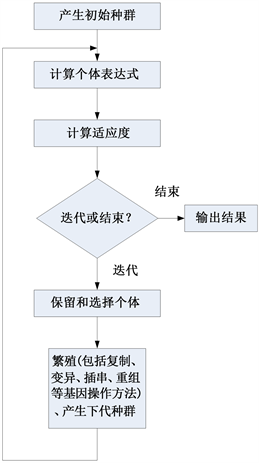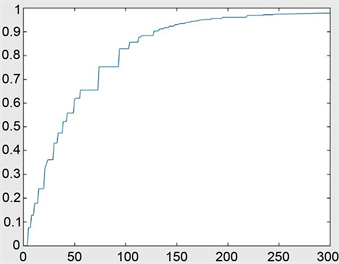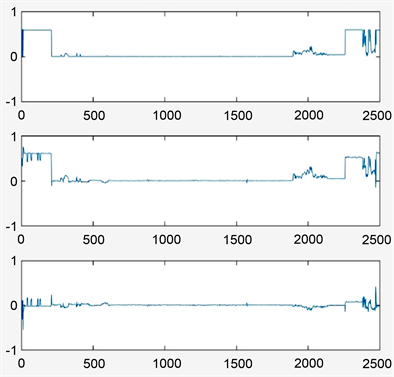# 基于基因表达式算法的某型发动机可调放气阀门基线建模Baseline Modeling of Engine Variable Bleed Valve Based on Gene Expression Programming Algorithm

DOI: 10.12677/CSA.2019.93064, PDF, HTML, XML, 下载: 427  浏览: 3,921

Abstract: Aiming at the problem that it is difficult to obtain the characteristic index of engine operation valves, adopting gene expression programming algorithm (GEP), using the quick access recorder (QAR) data, this paper mines and analyzes the engine variable bleed valves (VBV) operation base-line. The results show that, during VBV nonlinear regression analysis, GEP has the advantages of little deviation and high precision. GEP can also get specific and convenient function relations, which can improve the accuracy of VBV monitoring and fault diagnosis effectively.

1. 引言

2. 基因表达式编程算法Figure 1. GEP algorithm flowchart

3. VBV工作基线函数的GEP算法设计

$VBV=f\left(N1K12,N2K25,VSV,TAT,T2.5\right)$ (1)Table 1. Parameters of GEP algorithmFigure 2. Variation curve of fitnessFigure 3. The original measured curve, fitting curve and their errors

4. VBV工作基线函数曲线拟合结果分析

GEP算法以一台发动机的数据经过300代的迭代计算后，确定的VBV工作基线为：

$VBV=0.2957+1.15|{d}^{\frac{4}{3}}|+0.2a{d}^{2}+0.6714d+0.1126a+0.0043b+0.0049c+0.0047e$ (2)

$RMSE=\sqrt{MSE}=\sqrt{\frac{1}{n}\underset{i=1}{\overset{n}{\sum }}{\left({\stackrel{^}{y}}_{i}-{y}_{i}\right)}^{2}}$ (3)

Table 2. RMS error obtained GEP method

5. 结论

  Ferreira, C. (2017) Gene Expression Programming: A New Adaptive Algorithm for Solving Problems. Computer Science, 21, 87-129.  Ferreira, C. (2002) Gene Expression Programming: Mathematical Modeling by an Artificial Intelligence. Engineering Applications of Artificial Intelligence, 1, 223-225.  朱耀春. 基于基因表达式编程技术的非线性系统辨识研究[D]: [博士学位论文]. 北京: 华北电力大学, 2008.  元昌安. 基于GEP函数发现的智能模型库关键技术研究[D]: [博士学位论文]. 成都: 四川大学, 2006.  Luo, H., Wang, Y.R. and Cui, J. (2011) A Vague Description Method for Analog Circuit Fault Diagnosis Based on Description Sphere. Chinese Journal of Aeronautics, 24, 768-776. https://doi.org/10.1016/S1000-9361(11)60091-5  李冬, 宋岩, 马力, 等. 基于粗糙集和支持向量数据描述的发动机视情维修研究[J]. 燃气轮机技术, 2013, 26(1): 46-50, 58.  王欢. 涡扇发动机执行机构建模与控制方法研究[D]: [硕士学位论文]. 南京: 南京航空航天大学, 2012.  钟诗胜, 崔智全, 付旭云. Rolls-Royce发动机基线挖掘方法[J]. 计算机集成制造系统, 2010, 16(10): 2265-2270.  林兆福, 范作民. 发动机基线方程的建立和应用[J]. 中国民航大学学报, 1992(4): 20-32.  孔成安, 李文华, 尹湛. 利用QAR数据实施飞机性能监控[J]. 中国民用航空, 2008(10): 54.